## Sunday, February 28, 2010

### Series CircuitsThe current flows through each bulb/resistor is the same
I = I1 = I2

The potential difference across each resistor depends directly on its resistance. The potential difference supplied by the dry cells is shared by all the resistors.
V = V1 + V2

where V is the potential difference across the battery

If Ohm’s law is applied separately to each resistor, we get :
V = V1 + V2
IR = IR1 + IR2

If each term in the equation is divided by I, we get the effective resistance
R = R1 + R2

Worked example :There are two resistors in the circuit shown. Resistor R1 has a resistance of 1Ω. If a 3V voltage causes a current of 0.5A to flow through the circuit, calculate the resistance of R2.

solution :
V = IR
3=0.5(1+R2)
R2 = 5Ω

## Wednesday, February 24, 2010

The resistance of a conductor is a measure of the ability of the conductor to resist the flow of an electric current through it.
An important eﬀect of a resistor is that it converts electrical energy into other forms of energy,
such as heat and light.

Factors that affect resistance of a conductor:
1. the length of the conductor
~The longer the conductor , the higher its resistance

2. the cross-sectional area of the conductor
~The bigger the cross-sectional area, the lower the its resistance

3. type of material of the conductor
~ Different conductors with the same physical conditions have different resistance

4. the temperature of the conductor

~ The higher temperature of conductor , the higher the resistance.

simulation :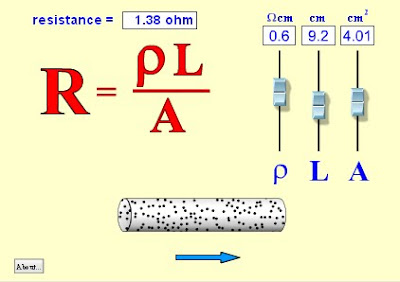R= resistance [Ω]
ρ = resistivity of the substance[Ωm]
L = length [m]
A = cross-sectional area of conductor [m 2]

Worked example :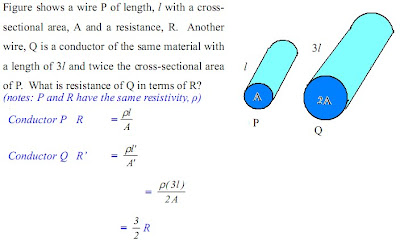## Monday, February 22, 2010

### Ohm’s Law

Ohm’s law states that the electric current, I flowing through a conductor is directly proportional to the potential difference across the ends of the conductor , if temperature and other physical conditions remain constant.

Mathematically, Ohm’s Law is written: V = IR

where
V = potential difference [V]
I = current [A]
R = resistance [Ω]

Ohm’s Law tells us that if a conductor is at a constant temperature, the voltage across the
ends of the conductor is proportional to the current. This means that if we plot voltage on the
y-axis of a graph and current on the x-axis of the graph, we will get a straight-line. The
gradient of the straight-line graph is then the resistance of the conductor.The resistance, R is a term that describes the opposition experienced by the electrons as they flow in a conductor. It is also defined as the ratio of the potential difference across the conductor to the current, I flowing through the conductor.

Non-ohmic conductors

Non-ohmic conductors are conductors which do not obey Ohm’s Law.
E.g., a light bulb whose resistance increases over time due to temperature increase (heating effect of current)
Note: In this whole chapter, all conductors and light bulbs are assumed to follow Ohm’s Law. There are no non-ohmic problems at SPM level.In general non-ohmic conductors have plots of voltage against current that are curved,
indicating that the resistance is not constant over all values of voltage and current.

Worked example :
Question :
What is the value of the resistor in the figure, if the dry cells supply 2.0 V and the ammeter reading is 0.5 A?

Solution :
V = IR
2.0 = 0.5 (R)
R = 4 Ω

Simulation

## Thursday, February 18, 2010

### Potential differenceGravitational potential energy at x is greater than the gravitational potential energy at y.
The ball will fall from x to y when the apple is released. This due to the difference in the gravitational energy.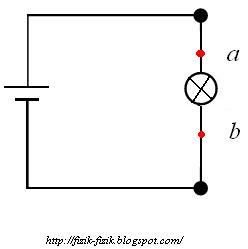Electric current flows from a to b, passing the bulb in the circuit and lights up the bulb.
This is due to the electric potential difference between the two terminals.
As the charges flow from a to b, work is done when electrical energy is transformed to light and heat energy.

Potential difference: Work done to move 1 Coulomb of charge from one point to another in an electric field.where V = potential difference [Volt]
E = energy released / work done [Joule]
Q = charge [Coulomb]

• Charges move from high potential to low potential, the moving charge called ”current”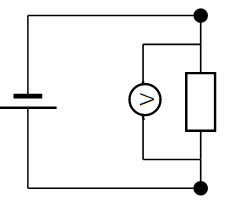• Potential difference can be measured with a voltmeter connected in parallel across two points within an electric circuit

Worked example :
1. If a charge of 5.0 C flows through a wire and the amount of electrical energy converted into heat is 2.5 J. Calculate the potential differences across the ends of the wire.

solution :

W = QV
2.5 = 5.0 (V)

V = 0.5 V

## Thursday, February 11, 2010

### 恭喜发财## Tuesday, February 9, 2010

### Electric fields

An electric field is the region in which an electric charge experiences a force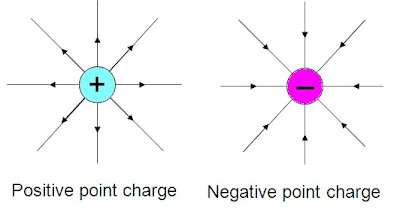Activity to observe the pattern of electric fields: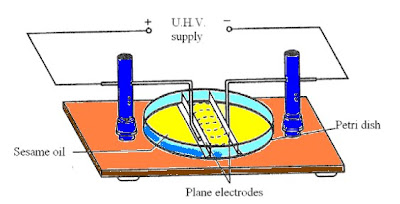When the power supply is turned on, the talcum powder will map the electrical field lines.
• Sesame oil is used because it conducts electricity only at extremely high potential difference
• The density of the field lines represent the intensity of the electric field
Simulation : http://www.vias.org/simulations/simusoft_efield.html

Natural phenomena == Lightning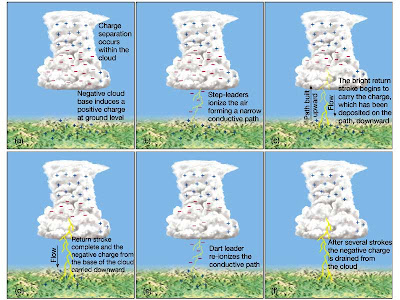IMAGE SOURCE http://www.ux1.eiu.edu/~jpstimac/1400/shockinglecture.html

During cloud formation, the friction of movement creates a lot of excess charge. This induced positive charges on the objects below it.
If the forces of attraction are strong enough, the negative charges in the clouds will be attracted towards the positive charges of the objects, resulting in lightning.

## Sunday, February 7, 2010

### ElectrostaticsAll objects surrounding us contain large amounts of electric charge.
There are two types of electric charge: positive charge and negative charge.
If the same amounts of negative and positive charge are brought together, they neutralise each other and there is no net charge.
Neutral objects are objects which contain positive and negative charges, but in equal numbers. However, if there is a little bit more of one type of charge than the other on the object then the object is said to be electrically charged.

The force exerted by non-moving (static) charges on each other is called the electrostatic force.
The electrostatic force between:
• like charges is repulsive
• opposite (unlike) charges is attractive.

Summery so far : There are two types of charges:
• Positive charge
• Negative charge
• Like charges repel whereas opposite charges attract.

Notes :
• Stationary electric charges are known as static electricity
• Electrostatics is the study of static electricity

## Friday, February 5, 2010

### Interference equation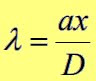where λ = wavelength [m]
a = distance between sources [m]
x = distance between two successive antinodal/nodal lines [m]
D = distance between a and x [m]

Quiz:

1. When coherent sound waves propagate from two loudspeakers of 2 m apart, an observer can hear the loudest sound. The loudspeakers are connected to a source waves with a frequency of 500 Hz and observer walks a distance of 10 m in front of the loudspeakers. If the speed of sound is 300 ms-1, what is the distance between two consecutive loudest sounds heard by the observer?

ans : 3 m

### Nodes and antinodes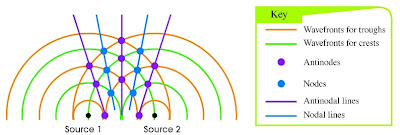Sources that produce waves of the same frequency and have a constant phase difference are called coherent sources.

Points of constructive interference are called antinodes (purple point) . The imaginary lines that connect the antinodes are called antinodal line. ( purple lines)

Points of destructive interference are called nodes. (blue point), whereas the nodes are connected by the nodal lines. (blue lines)

## Wednesday, February 3, 2010

### Interference of waves

When two or more waves meet, they superpose or combine at a particular point. The waves are said to interfere. This phenomenon is called interference.When two pulses meet, they superpose. The resulting pulse has a bigger amplitude which is equal to the vector sum of the amplitudes of the individual pulses. This is called constructive interference.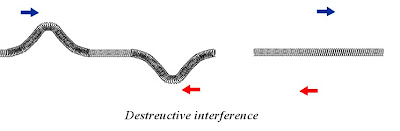If the pulses that combine are same amplitude but are out of phase, the resultant amplitude is zero. This is called destructive interference.

## Monday, February 1, 2010

### The principle of superposition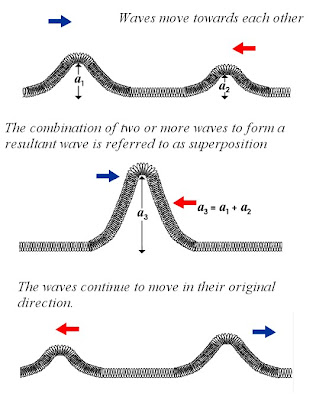The principle of superposition states that the when two waves meet at a certain point, the resultant displacement at that point is the vector sum of the individual waves.

After waves pass through each other, each waves continues along its original direction of travel, and their original amplitudes remain unchanged.